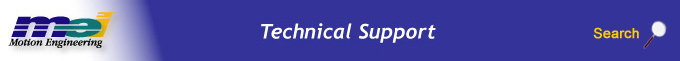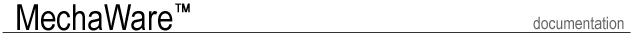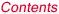.Select a Step ......................................................... Introduction Features User Interface Requirements Types of MechaWare Blocks Block Simulink Library Matlab Utility Software Tools Case Study Tutorial

Matlab Utility

M-Files

C:\MEI\MechaWare\matlab14\Utilities\Lowpass.m
C:\MEI\MechaWare\matlab14\Utilities\MultiStageBIQ.m
C:\MEI\MechaWare\matlab14\Utilities\MWOBS.m
C:\MEI\MechaWare\matlab14\Utilities\Notch.m
C:\MEI\MechaWare\matlab14\Utilities\PID2BIQ.m
C:\MEI\MechaWare\matlab14\Utilities\Resonator.m
C:\MEI\MechaWare\matlab14\Utilities\UnityGain.m
C:\MEI\MechaWare\matlab14\Utilities\ZP2BIQ.m

LowPass

The LowPass Matlab utility program is used to make the MechaWare structure required by Simulink. It generates the text file that is downloaded to the XMP/ZMP for a low pass filter specified in the LowPass utility arguments by using the standard second order Biquad block. The low pass filter type is a second order Butterworth Design.

 Matlab Command Line BIQ=Lowpass (Hertz, Ts, name) Input Argument Description Hertz Filter Break Point in Hertz Ts Controller Sample Rate name Name of Parameter text file. This should be the same name as the output variable. Output Variable Output Variable BIQ Name of MechaWare structure used by Simulink. Example BIQ=Lowpass (250, Ts, 'BIQ')MultiStageBIQ

The Matlab utility program is used to make the MechaWare structure required by Simulink. It also generates the text file that is downloaded to the XMP/ZMP motion controller for a multistage-cascaded biquad filter.

 Matlab Command Line [BIQ]=MultiStageBIQ (b, a. Ts, blockname) Input Argument Description b Numerator of Z-domain transfer function. a Denominator of Z-domain transfer function. Ts Controller Sample Rate blockName Name of Parameter text file. This should be the same name as the output variable. Output Variable Output Variable BIQ Name of MechaWare structure used by Simulink.

MWOBS

The Matlab utility program is used to make the MechaWare structure required by Simulink. It also generates the text file that is downloaded to the XMP/ZMP motion controller for the Observer Block.

 Matlab Command Line [OBS]=MakeEcaOBS (Obs, blockname) Input Argument Description Obs The state space description of the observer block in discrete time. Mechaware Observers are 2 input, 1 output, Nth order systems. Obs is a Matlab LTI (Linear Time Invariant) Structure. blockName Name of Parameter text file. This should be the same name as the output variable. Output Variable Output Variable OBS Name of MechaWare structure used by Simulink.

Notch

The Matlab utility program is used to make a second order notch filter structure required by Simulink. It also generates the text file that is downloaded to the XMP/ZMP motion controller. The low pass filter type is a second order Butterworth design transformed to a notch filter.

 Matlab Command Line BIQ=Notch (Hertz,BW,Ts,name) Input Argument Description Hertz Notch Center Frequency in Hertz BW Notch Width in Hertz Ts Controller Sample Rate name Name of Parameter text file. This should be the same name as the output variable. Output Variable Output Variable BIQ Name of MechaWare structure used by Simulink. Example BIQ=Notch(100,100,Ts,'BIQ')PID2BIQ

The Matlab utility program is used to make the MechaWare structure required by Simulink. It also generates the text parameter file that is downloaded to the XMP/ZMP motion controller. PID2BIQ implements a PID algorithm using the standard second order Biquad block: pid2biq=PID2BIQ(Ypid,name)

 Matlab Command Line BIQ=Notch (Hertz,BW,Ts,name) Input Argument Description Ypid A matlab structure that defines pid gains and sample rate. The structure has the following elements:      Ypid.Kp      Ypid.Ki      Ypid.Kd      Ypid.Ts name Name of Parameter text file. This should be the same name as the output variable. Output Variable Output Variable BIQ Name of MechaWare structure used by Simulink. Example ypid =      Kp: 1      Ki: 0.5000      Kd: 50      Ts: 1.2500e-004 BIQ=PID2BIQ(ypid,'BIQ')Resonator

The Matlab utility program is used to make the mechaware structure required by Simulink. It also generates the text parameter file that is downloaded to the XMP/ZMP motion controller for a Resonator Filter using the standard second order Biquad block.

 Matlab Command Line BIQ=Resonator ( fn,bw,depth,Ts,name ) Input Argument Description fn Center Frequency in Hertz bw Width in Hertz depth Depth or Height of filter Magnitude in dB. Ts Controller Sample Rate name Name of Parameter text file. This should be the same name as the output variable. Output Variable Output Variable BIQ Name of MechaWare structure used by Simulink. Example BIQ=Resonator(100,100,20,Ts,'BIQ')BIQ=Resonator(100,100,-20,Ts,'BIQ')UnityGain

The Matlab utility program is used to make the MechaWare structure required by Ssimulink. It also generates the text parameter file that is downloaded to the XMP/ZMP motion controller for a UnityGain Filter. It uses the standard second order Biquad block.

 Matlab Command Line BIQ=UnityGain (Ts, name) Input Argument Description Ts Controller Sample Rate name Name of Parameter text file. This should be the same name as the output variable. Output Variable Output Variable BIQ Name of MechaWare structure used by Simulink. Example BIQ=UnityGain(Ts,’BIQ’)

ZP2BIQ

The Matlab utility program is used to make the MechaWare structure required by Simulink. It also generates the text parameter file that is downloaded to the XMP/ZMP motion controller for a second order filter specified with 2 poles, 2 zeros, and DC gain. using standard the 2nd order Biquad block.

 Matlab Command Line BIQ=UnityGain (Ts, name) Input Argument Description z Transfer Function zeros in Hertz;      z is a matlab array      z=[z1;z2] p Transfer Function poles in Hertz;      p is a matlab array      p=[p1;p2] k DC gain of Transfer Function Ts Controller Sample Rate name Name of Parameter text file. This should be the same name as the output variable. Output Variable Output Variable BIQ Name of MechaWare structure used by Simulink. Example BIQ=ZP2BIQ([-80; -100],                     [-20 ;-1000],                     10,                     Ts,                     'BIQ')MATLAB Analysis Files

Calculates and plots the Frequency Response transer funtion of Y(s)/U(s) of two input vecotrs: u and y.

fftcalc uses Welch's Method to calculate the frequency response.

 Matlab Command Line [P,F]=fftcalc(u, y, Fs, Measpar); Input Argument Description u Input data vector y Output vector Fs Corresponding Sample Rate for input and output data Measpar Matlab structure that defines FFT and plotting parameters. Measpar,NFFT Number of points in FFT. Measpar. WINDOW Window vector used for analysis has same number of points as NFFT. Measpar,OVERLAP Overlap specified as number of points. Measpar,Fs Sample Rate of Data Collection Measpar.MagRange Vector [min max], containing minimum and maximum values plotted on the magnitude plot. Measpar.PhasRange Vector [min max], containing minimum and maximum values plotted on the phase plot. Measpar.FreqRange Vector [min max], containing minimum and maximum values that Frequency is plotted. Output Variable Output Variable P Complex Frequency Response Fs Corresponding Frequency in Hertz for P Example [P,F]=fftcalc(u,y,Fs,measpar); measpar =      NFFT: 8192      WINDOW: [8192x1 double]      OVERLAP: 4096            Fs: 8000            MagRange: [0.0100 100]            PhasRange: [-180 180]            FreqRange: [1 1000]

 Legal Notice  |  Tech Email  |  Feedback        Copyright © 2001-2009 Motion Engineering